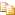﻿Round Method (value, pixelSize)AvalonEdit
Round Method (value, pixelSize)
NamespacesICSharpCode.AvalonEdit.UtilsPixelSnapHelpersRound(Double, Double)
Rounds value to a whole number of pixels.Declaration Syntax
 C# Visual Basic Visual C++
```public static double Round(
double value,
double pixelSize
)```
```Public Shared Function Round ( _
value As Double, _
pixelSize As Double _
) As Double```
```public:
static double Round(
double value,
double pixelSize
)```Parameters
value (Double)
pixelSize (Double)

Assembly: ICSharpCode.AvalonEdit (Module: ICSharpCode.AvalonEdit.dll) Version: 5.0.0.4238